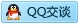# python 正则表达式扩展表示

2020-01-15 11:09 1186 查看

### python 正则表达式扩展表示

• 二、匹配不用保存的分组；
• 三、
• 四、(?#***)：表示注释，所有内容都被忽略；
• 五、
• 六、(?(id/name)yes-pattern|no-pattern)：
• 七、正则替换，sub：
• # 一、(?iLmsux)：在正则表达式中嵌入一个或者多个特殊“标记”参数，可多个一起用；

### 3、(?m)：表示 re.M/MULTILINE，实现多行混合，data 数据多行；

```import re
result = re.findall(r'(?im)(^th[\w\s]+)', '''
This line is the first,
another line,
that line, it's the best
''')
print(result) # ['This line is the first', 'that line']```

### 4、(?s)：表示符号 . 可以代表任意符号（正常情况符号 . 表示除 \n 之外的任意符号）；

```import re
result = re.findall(r'(?s)th.+', '''
This line is the first,
another line,
that line, it's the best
''')
print(result)# ["the first,\nanother line,\nthat line, it's the best\n"]```

### 6、(?x)：表示允许用户通过抑制在正则表达式中使用空白符（除了在字符类中或者反斜线转义中）来创建更易读的正则表达式，正则表达式多行；# 二、匹配不用保存的分组；

```result = re.findall(r'http://(?:\w+\.)*(\w+\.com)', 'http://google.com http://google.com http://code.google.com')

# 三、

### 1、(?P***)：像一个仅由 name 标识而不是数字 ID 标识的正则分组匹配；

```result = re.search(r'\((?P<areacode>\d{3})\) (?P<prefix>\d{3})-(?:\d{4})', '(800) 555-1212').groupdict()
print(result)# {'areacode': '800', 'prefix': '555'}```

### 2、(?P=name)：在同一个字符串中，匹配由 (?😛***) 分组的之前文本；# 五、

### 1、(?=***)：如下例1，匹配后面是 \d+ 的 [a-z]+；

```data = 'a1b2ce3@4.5d_6f'
re.findall(r'[a-z]+(?=\d+)', data) # ['a', 'b', 'ce']
re.findall(r'\d+(?=[a-z]+)', data) # ['1', '2', '5', '6']```### 2、(?!***)：如下例1，匹配后面不是 \d+ 的 [a-z]+；

```re.findall(r'[a-z]+(?!\d+)', data) # ['c', 'd', 'f']
re.findall(r'\d+(?![a-z]+)', data) # ['3', '4']```### 3、(?<=***)：如下例1，匹配前面是 \d+ 的 [a-z]+；

```re.findall(r'(?<=\d)[a-z]+', data) # ['b', 'ce', 'd', 'f']
re.findall(r'(?<=[a-z])\d+', data) # ['1', '2', '3']```

### 4、(?<!***)：如下例1，匹配前面面不是 \d+ 的 [a-z]+；

```re.findall(r'(?<!\d)[a-z]+', data) # ['a', 'e']
re.findall(r'(?<![a-z])\d+', data) # ['4', '5', '6']```

# 六、(?(id/name)yes-pattern|no-pattern)：

解释：先匹配 id 或者 name 对应的分组，如果能匹配到，就继续匹配 yes-pattern；如果不能匹配到，就继续匹配 no-pattern；

重点：group 不允许为空的时候（即 group 后面无 ？），group 对目标字符串匹配失败时，整体匹配失败，此时 no-pattern 部分自然失效；所以想要特殊规则 no-pattern 部分生效，判断条件 group 匹配数可以为0，即 group 后面必须加 ？ 或者 如下例加一个没分组的group，直接用 no-pattern 部分；

可以仅匹配 group 部分，如果 yes-pattern 和 no-pattern 没有匹配到，则会输出 group 匹配的内容；

## 能匹配 no-pattern 的情况：

### 1、？ 或者 *：

```re.search(r'([a-z]+)(?(1)\.|\w+)', 'hujinqun123@qq.com')# <_sre.SRE_Match object; span=(12, 15), match='qq.'>
re.search(r'([a-z]+)(?(1)@|\w+)', 'hujinqun123@qq.com')# None
re.search(r'([a-z]+)?(?(1)@|\w+)', 'hujinqun123@qq.com')# <_sre.SRE_Match object; span=(0, 11), match='hujinqun123'>```

### 2、(?😦…)| …) ：取消分组符号里面有分组和没分组的情况，| 隔开，分组匹配失败就直接用未分组的那个匹配 no-pattern部分：

下面两个表达方式是一样的，只能匹配 ‘xy’和‘yx’：

```re.search(r'(?:(x)|y)(?(1)y|x)', 'yx')# <_sre.SRE_Match object; span=(0, 2), match='yx'>
re.search(r'(?:(?P<aaa>x)|y)(?(aaa)y|x)', 'yx'))```
`re.search(r'(?i)(?:(?P<first>a)|b)(?(first)b|c)', 'AaCBc')# <_sre.SRE_Match object; span=(3, 5), match='Bc'>`

说明：
1、(?i)：忽略大小写；
2、(?: *** )：取消保存分组，意味着后面的分组只有 first（a）；
3、|：表示两个正则表达式或者；
4、first 分组（a）能匹配到，匹配 yes-pattern 部分，字符串中无’ab’，该匹配返回 None；
5、然后 b 来匹配，此时不存在 group，直接匹配 no-pattern 部分，‘bc’，匹配成功，返回 ‘Bc’；

```re.search(r'(?i)(?:(?P<first>a)|b)?(?(first)b|c)', 'AaCBc')# <_sre.SRE_Match object; span=(2, 3), match='C'>
re.search(r'(?i)(?:(?P<first>a)?|b)(?(first)b|c)', 'AaCBc')# <_sre.SRE_Match object; span=(2, 3), match='C'>```

说明：步骤1,2,3,4同上；
5、上一步返回 None，由于分组group 匹配数可以为 0，直接匹配 no-pattern 部分，‘c’，匹配成功，返回‘C’；

### 3、对比1：

```re.search(r'(?P<first>a)(?(first)b|c)', 'aab')# <_sre.SRE_Match object; span=(1, 3), match='ab'>
# 说明：先匹配 first 分组（对应 a），能匹配到，结果 ab；

re.search(r'(?P<first>a)(?(first)b|c)', 'aacb')# None
# 说明：先匹配 first 分组（对应 a），能匹配到，再匹配 ab，匹配失败，返回 None；

re.search(r'(?P<first>a)?(?(first)b|c)', 'aac')# <_sre.SRE_Match object; span=(2, 3), match='c'>
# 说明：分组 first 可有可无的情况，先匹配 first 分组（对应 a），能匹配到，再匹配 ab，匹配失败，分组 first 不存在的情况，结果 c；

re.search(r'(\d+)?(?(1)\w+|pythontab\.\w+)', 'pythontab.com')# <_sre.SRE_Match object; span=(0, 13), match='pythontab.com'>

re.search(r'(\d+)?(?(1)\w+)', '100pythontab')# <_sre.SRE_Match object; span=(0, 12), match='100pythontab'>
# 说明：如果不想匹配 no-pattern 部分，可以连同 | 符号一起不写；

re.search(r'(\d+)(?(1)\w+|pythontab\.\w+)', 'pythontab.com')# None
# 说明：整体规则匹配失败，返回空，no-pattern 部分并未执行；

re.search(r'(\d+)(?(1)\w+|pythontab\.\w+)', '1pythontab.com')# <_sre.SRE_Match object; span=(0, 10), match='1pythontab'>
# 说明：group 和 yes-pattern 部分匹配到了；

re.search(r'(\d+)?(?(1)\w+|pythontab\.\w+)', 'pythontab')# None
# 说明：如果 group 没有匹配到，且它可有可无，那么 group 会被认为匹配不成功，继续执行 no-pattern 部分；只有是可有可无的情况下，才有可能执行 no-pattern 部分；

re.search(r'(\d+)(?(1)\w+|pythontab\.\w+)', '1000')# <_sre.SRE_Match object; span=(0, 4), match='1000'>
# 说明：只匹配 group 部分，yes-pattern 部分没有匹配；```

# 七、正则替换，sub：

re.sub(pattern, repl, string, conunt=0, flags=0)

```参数说明：

必选参数：pattern, repl, string
可选参数：count, flags

①、pattern：表示正则表达式中的模式字符串；
②、repl：可以是字符串，也可以是函数；
a、如果 repl 是字符串的话，其中任何反斜杠转义字符，都会被处理的；此时需要指定前缀 r，才是 pattern；
\n：换行符；\r：回车符；\g<name>：对应命了名的组，named group；其他不能识别的就被处理为字母本身；
b、函数的情况；
③、表示要被处理，要被替换的那个 string 字符串；
④、替换次数，即只处理前 count 个匹配到的内容；
⑤、类似：flags=re.I；```
```inputstr = 'hello 123 world 456'
intstr = matched.group()
return str(int(intstr) + 2)

print(re.sub(r'\d+', add, inputstr)) # hello 125 world 458
print(re.sub(r'\d+', lambda matched:str(int(matched.group()) + 3), inputstr)) # hello 126 world 459
print(re.sub('[a-z]+', lambda matched:matched.group()+'hjq', inputstr)) # hellohjq 123 worldhjq 456

data = '2019-06-29'
# sub：替换；第一个参数 pattern， 第二个参数 需要替换成的字符， 第三个参数 字符原串
re.sub(r'-', '/', data)# 2019/06/29
re.sub(r'(\d{4})-(\d{2})-(\d{2})', r'\1++\3##\2', data) # 2019++29##06
re.sub(r'(?P<year>\d{4})-(?P<month>\d{2})-(?P<day>\d{2})', r'\g<month>/\g<day>/\g<year>', data) # 06/29/2019```
```result = re.sub(r'\((?P<areacode>\d{3})\) (?P<prefix>\d{3})-(?:\d{4})',
'(\g<areacode>) \g<prefix>-xxxx',
'(800) 555-1212')
print(result)# (800) 555-xxxx

old_name = 'com.tencent.tmgp.hjq'
old_name.replace('.', '\\') # com\tencent\tmgp\hjq
re.sub(r'\.', r'\\', old_name) # com\tencent\tmgp\hjq

s = '1234567890'
# 此处 \1 \2 \3 表示的pattern中的三个分组
# \1：表示3 \3：表示6 \2：表示3和6中间的数字浪子哥学习笔记 发布了13 篇原创文章 · 获赞 0 · 访问量 124 私信 关注
内容来自用户分享和网络整理，不保证内容的准确性，如有侵权内容，可联系管理员处理